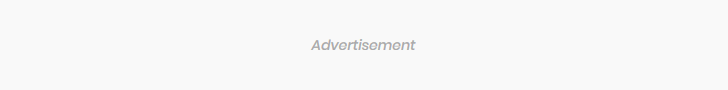### Subscribe Us# Application of exponent (CIE)

$\def\D{\displaystyle}$

1 (CIE 2012, s, paper 12, question 9)
Variables $\D N$ and $\D x$ are such that $\D N = 200 + 50e^{\frac{x}{100}}.$
(i) Find the value of $\D N$ when $\D x = 0.$ 
(ii) Find the value of $\D x$ when $\D N = 600.$ 
(iii) Find the value of $\D N$ when $\D \frac{dN}{dx}=45.$ 

2 (CIE 2014, w, paper 21, question 5)
The number of bacteria $\D B$ in a culture, $\D t$ days after the first observation, is given by
$B= 500 +400e^{0.2t}.$
(i) Find the initial number present. 
(ii) Find the number present after 10 days. 
(iii) Find the rate at which the bacteria are increasing after 10 days. 
(iv) Find the value of $\D t$ when $\D B = 10000.$ 

3 (CIE 2014, w, paper 23, question 4)
The profit \\D P$made by a company in its nth year is modelled by $P=1000e^{an+b}.$ In the first year the company made \$2000 profit.
(i) Show that $\D a + b = \ln 2.$ 
In the second year the company made \$3297 profit. (ii) Find another linear equation connecting$\D a$and$\D b.$ (iii) Solve the two equations from parts (i) and (ii) to find the value of$\D a$and of$\D b.$ (iv) Using your values for$\D a$and$\D b,$find the profit in the 10th year.  4 (CIE 2016, w, paper 21, question 4) The number of bacteria,$\D N,$present in a culture can be modelled by the equation$\D N= 7000+ 2000e^{-0.05t},$where$\D t$is measured in days. Find (i) the number of bacteria when$\D t = 10,$ (ii) the value of$\D t$when the number of bacteria reaches 7500,  (iii) the rate at which the number of bacteria is decreasing after 8 days.  5 (CIE 2017, march, paper 12, question 11) It is given that$\D y = Ae^{bx} ,$where$\D A$and$\D b$are constants. When$\D \ln y$is plotted against$\D x$a straight line graph is obtained which passes through the points (1.0, 0.7) and (2.5, 3.7). (i) Find the value of$\D A$and of$\D b.$ (ii) Find the value of$\D y$when$\D x = 2.$ 6 (CIE 2017, march, paper 22, question 2) The value,$\D V$dollars, of a car aged$\D t$years is given by$\D V = 12000 e^{-0.2t}.$(i) Write down the value of the car when it was new.  (ii) Find the time it takes for the value to decrease to$\D \frac{2}{3}$of the value when it was new.  7 (CIE 2017, s, paper 12, question 7) It is given that$\D y = A(10^{bx}),$where$\D A$and$\D b$are constants. The straight line graph obtained when$\D \lg y$is plotted against$\D x$passes through the points (0.5, 2.2) and (1.0, 3.7). (i) Find the value of$\D A$and of$\D b.$ Using your values of$\D A$and$\D b,$find (ii) the value of$\D y$when$\D x = 0.6,$ (iii) the value of$\D x$when$\D y = 600.$ 8 (CIE 2018, s, paper 11, question 5) The population,$\D P,$of a certain bacterium$\D t$days after the start of an experiment is modelled by$\D P = 800e^{kt},$where$\D k$is a constant. (i) State what the figure 800 represents in this experiment.  (ii) Given that the population is 20 000 two days after the start of the experiment, calculate the value of$\D k.$ (iii) Calculate the population three days after the start of the experiment.  9 (CIE 2018, s, paper 12, question 7) A population,$\D B,$of a particular bacterium,$\D t$hours after measurements began, is given by$\D B =1000e^{\frac{t}{4}}.$(i) Find the value of$\D B$when$\D t = 0.$ (ii) Find the time taken for$\D B$to double in size.  (iii) Find the value of$\D B$when$\D t = 8.$ 1.$\D 250;208;4700$2. (i)$\D 900$(ii)$\D 3456$(iii)$\D 591$(iv)$\D 15.8$3. (ii)$\D 2a + b = \ln 3.297$(iii)$\D a = 0.5; b = 0.193$(iv)$\D n = 10; P = 180000$4. (i)$\D 8213$(ii)$\D 27.7$(iii)$\D \pm 67$5. (i)$\D b = 2;A = 0.273,$(ii)$\D 14.9$6. (i)$\D 12 000$(ii)$\D 2$7. (i)$\D b=3,A=5.01$or$\D 10.7$(ii)$\D y=315$or$\D 102.5$(iii)$\D x = 0.693$8. The number of bacteria at the start of the experiment$\D 1.61,100 000$9. (i)$\D 1000,$(ii)$\D 2.77$(iii)$\D 7389\$